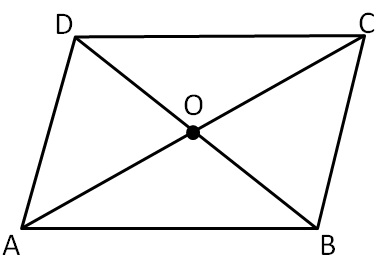Diagnols of parallelogram

Chapter 3 Class 8 Understanding Quadrilaterals
Concept wise

Let's consider parallelogram ABCDHere, Diagonals is the line joining opposite verices of the parallelogram. So, AC and BD are the diagonals

In a parallelogram,

Diagonals of parallelogram bisect each other

⇒ AO = CO and BO = DO

Learn in your speed, with individual attention - Teachoo Maths 1-on-1 Class